# 针对MPAndroidChart—3.0 X轴显示字符串类型文字的问题 以及一些bug 新人多多指教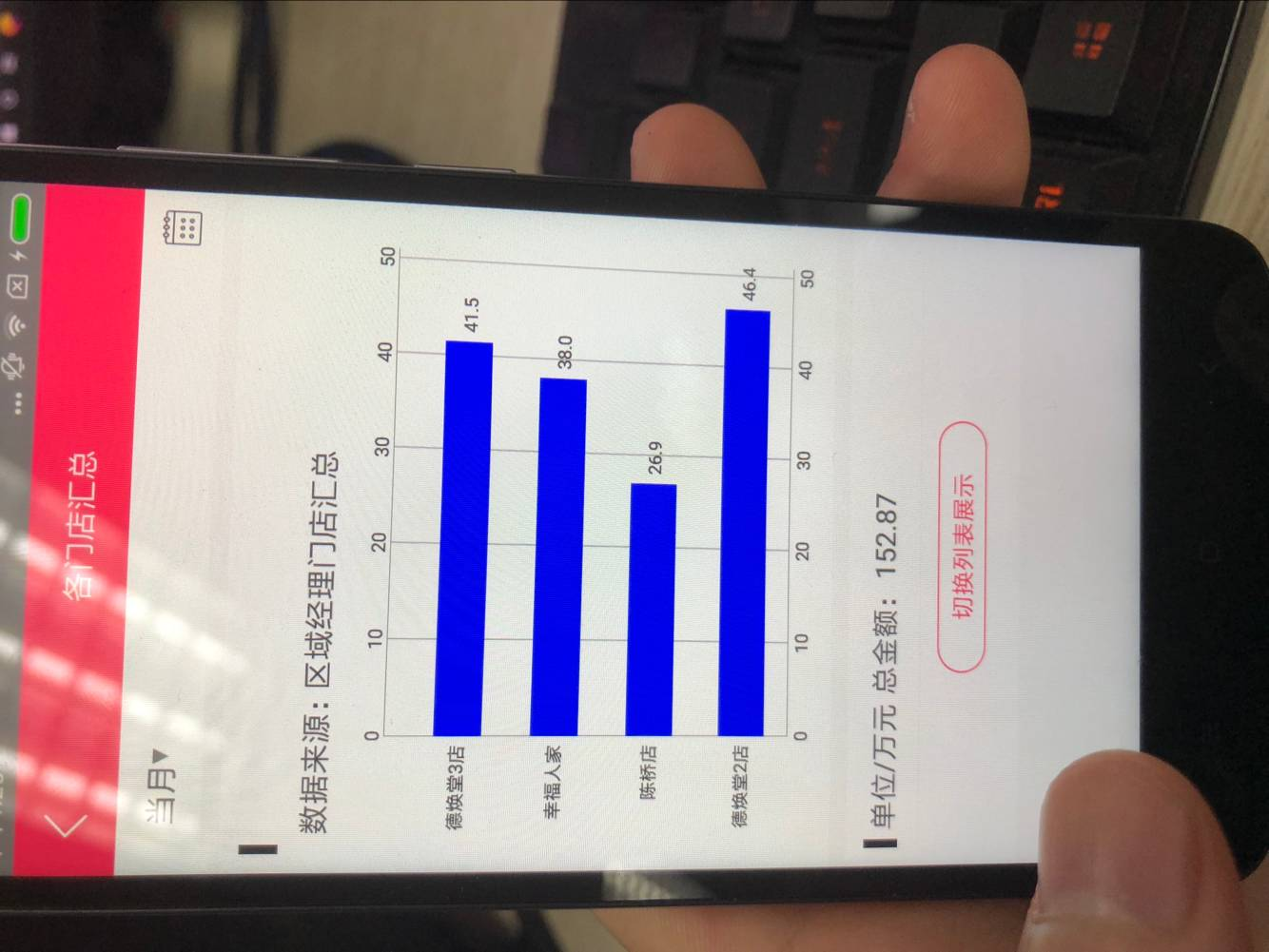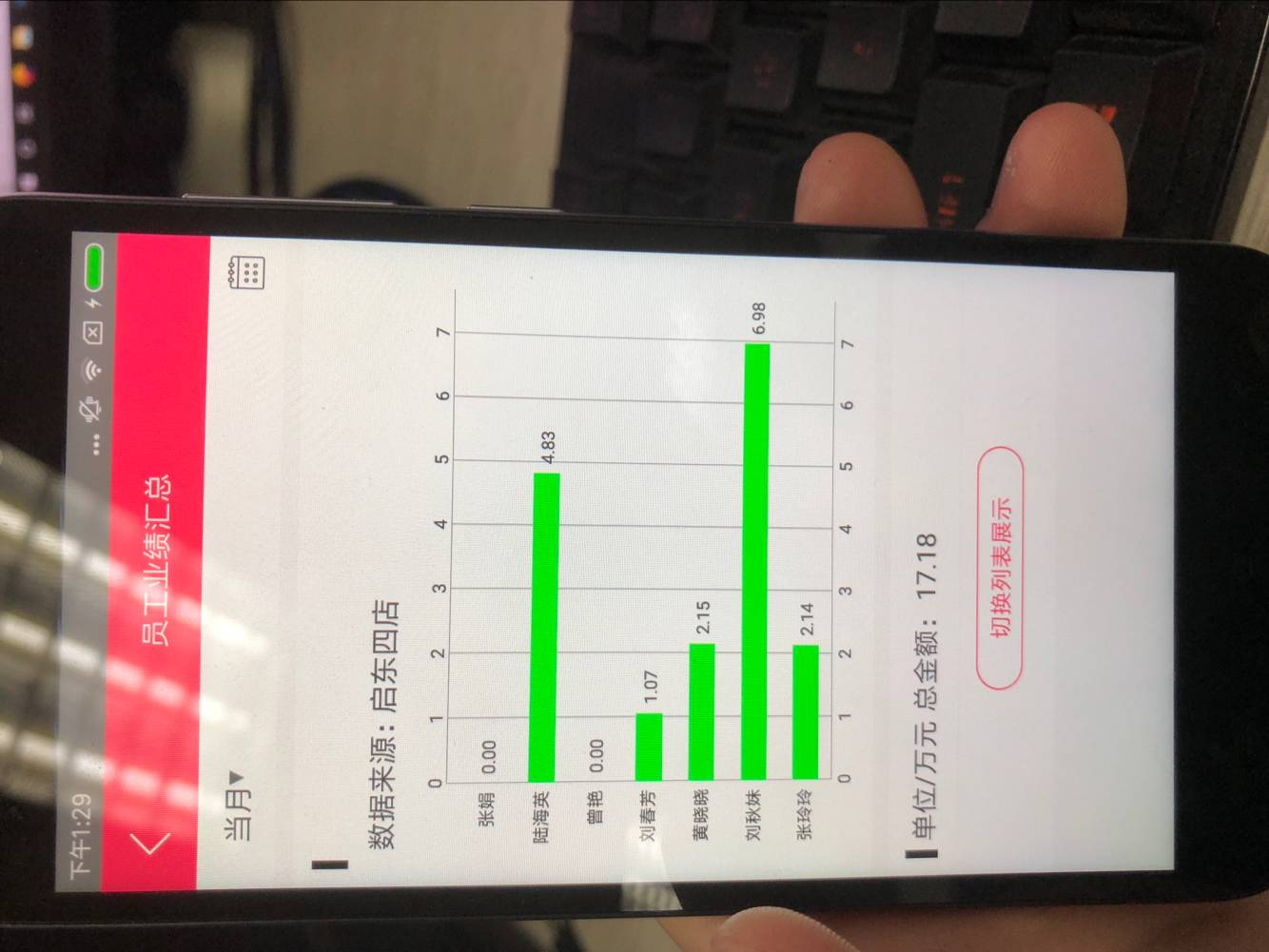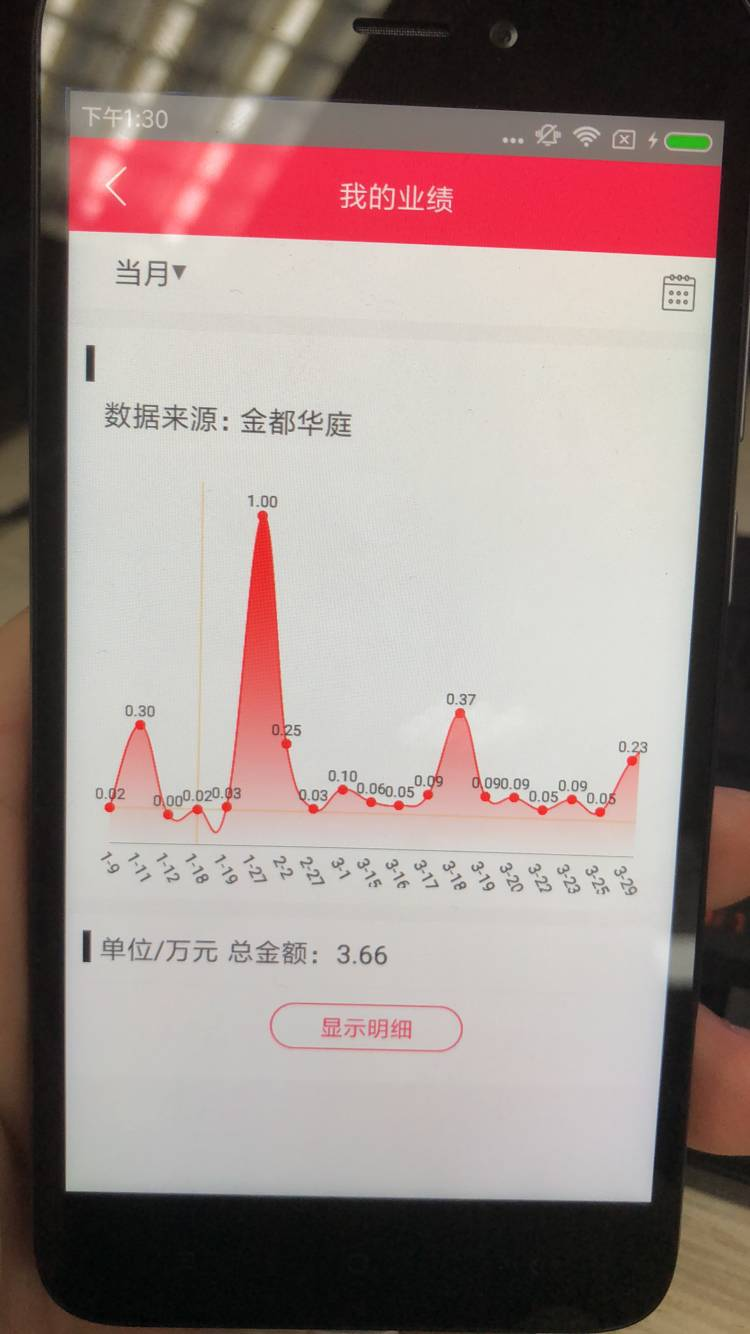HorizontalBarChart 这个横向柱状图 X轴的字符串问题 找了好久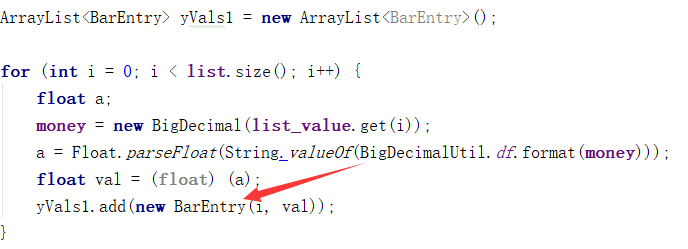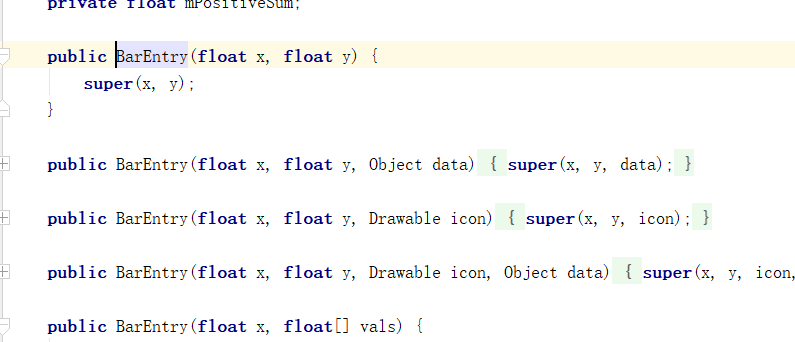class LabelFormatter implements IAxisValueFormatter {
private final String[] mLabels;
public LabelFormatter(String[] labels) {
mLabels = labels;
}
@Override
public String getFormattedValue(float value, AxisBase axis) {
try {
return mLabels[(int) value];
} catch (Exception e) {
e.printStackTrace();
return mLabels;
}
}
}

String[] str = new String[listtittle.size()];

for (int j = 0; j < listtittle.size(); j++){
str[j] = listtittle.get(j);
}

XAxis xAxis = mHorizontalBarChart.getXAxis();
LabelFormatter labelFormatter = new LabelFormatter(str);
xAxis.setValueFormatter(labelFormatter);

for (int i = 0; i < list.size(); i++) {
float a;
money = new BigDecimal(list_value.get(i));
a = Float.parseFloat(String.valueOf(BigDecimalUtil.df.format(money)));
float val = (float) (a);
}

BarDataSet set1;
set1 = new BarDataSet(yVals1, "DataSet 1");
set1.setColor(Color.GREEN);
ArrayList<IBarDataSet> dataSets = new ArrayList<IBarDataSet>();
BarData data = new BarData(dataSets);
data.setValueTextSize(10f);
data.setBarWidth(0.5f);
mHorizontalBarChart.setData(data);

 XAxis xl = mHorizontalBarChart.getXAxis();
xl.setPosition(XAxis.XAxisPosition.BOTTOM);
//            xl.setDrawAxisLine(true);
xl.setDrawGridLines(false);
xl.setGranularity(1f);
xl.setAxisMinValue(-0.5f);
xl.setLabelCount(listtittle.size(), false);